International Journal of Energy and Power Engineering
Volume 5, Issue 2, April 2016, Pages: 48-59

Thermal Analysis and Modelling of Thermal Storage in Solar Water Heating Systems

Samuel Sami1, Jorge Zatarain2

1Research Center for Renewable Energy, Catholic University of Cuenca, Cuenca, Ecuador

2Faculty of Energy Engineering, Universidad Politécnica de Sinaloa, Sinaloa, México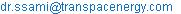(S. Sami)

Samuel Sami,Jorge Zatarain. Thermal Analysis and Modelling of Thermal Storage in Solar Water Heating Systems. International Journal of Energy and Power Engineering. Vol. 5, No. 2, 2016, pp. 48-59. doi: 10.11648/j.ijepe.20160502.14

Received: March 7, 2016; Accepted: March 13, 2016; Published: April 10, 2016

Abstract: A numerical mathematical model has been developed to predict the thermal behavior of phase change material during thermal storage in a thermal tank. The model is based upon energy conservation equations and includes fusion of the phase change material. The thermal behavior of the phase change material during charging and discharging have been studied numerically, and analyzed under different conditions. Comparisons were made against experimental data for validation purposes of the predictive model. The model fairly predicted experimental data obtained at various inlet conditions of the phase change material.

Keywords: Phase Change Material, Thermal Storage, Thermal Tank, Numerical Model, Simulation, Experimental Validation

Contents

1. Introduction

Solar collectors convert solar irradiation energy to thermal energy that is transferred to working fluid in solar thermal applications. The heat carried by the working fluid can be used to either provide domestic hot water/heating, or to charge phase change material in a thermal storage tank for heat supply during non-solar periods.

Excellent thermal properties such as high thermal storage capacity, good heat transfer rate between the heat storage material, heat transfer fluid and good stability to avoid chemical and mechanical degradation, are the key factors to viable solar thermal energy storage system [1-8].

Phase change materials (PCMs) can store/release significant amount of heat during melting/solidification phase change processes. PCM during sensible heat storage experiences a large temperature rise/drop when storing/releasing thermal energy. However, latent heat storage occurs at nearly isothermal way. This makes latent heat storage desirable and favourable for thermal storage applications. Paraffin waxes are widely used as heat of fusion storage materials due to their availability to store thermal energy in a large temperature range .

A comparison of five commercial paraffin waxes as latent heat storage materials have been presented by Ukraincyk et al. . The temperatures, heat capacities, of solid, and liquid paraffin waxes were measured by differential scanning calorimetry DCS. The thermal diffusivity was determined utilizing transient method.

An experimental investigation was carried out by Khot  to understand the improvement of thermal storage system using water-PCM in comparison with water. The experiments were conducted for the same heat input during accelerated conditions in both Water-PCM and also water inside thermal storage tank system.

Lin et al.  reported on an experimental investigation of solar water heater with thermal storage. In the study, an outdoor integrated phase change material flat plat solar collector was carried out with paraffin wax. The PCM gave the highest performance when considering the day and night time efficiency compared to the case without PCM.

Another study to investigate the characteristics of thermal energy storage in solar system using phase change paraffin wax in a thermal storage tank was presented by Kanimozhi . The tank was instrumented to measure inlet and outlet water temperature as well as water mass flow rate. The solar energy was absorbed and stored in PCM storage unit as latent heat.

Recently, another investigation has been presented by Kuolkarni and Deshmukh  to undertake a study of the feasibility of storing solar energy using phase change materials PCMs to heat water for domestic purposes during the night times. The experiment showed that with using PCM, cooling rate during the night decrease as well as efficiency and heat storage capacity increases. Hot water storage tank systems store sensible energy; however, this requires added weight. However, the study showed that using phase change materials PCMs solves this problem and can satisfy the night and day-time energy demand.

Milisic  in his thesis described the state of the art progress in applying PCM materials for energy storage in thermal tanks, and opportunities of their future applications. In addition, he presented a mathematical model consisted of charging process and discharging process. Furthermore, Muhmud et al.  presented a theoretical model to investigate the thermal and physical properties of a phase change material consisted of paraffin wax and 5% aluminum powder in solar air heater. The results showed that the air temperature gained was due to energy discharge process decease with increasing the air mass flow rate.

A mathematical model for describing the heat stored in thermal storage tank and phase change material behaviour in thermal solar tanks is presented hereby. The model was established after the energy conservation coupled with the heat transfer equations. In the following sections, simulations of a thermal solar tank using paraffin wax will be presented and analyzed. In addition, several experimental data are used in order to validate the mathematical model. The mathematical model, in particular, was used to study of the effect of operating conditions such as solar radiation, working fluid flow rates, initial working fluid and paraffin wax temperatures on the phase change material behaviour and system performance as well as the thermal conversion efficiency.

2. Mathematical Model

A schematic of the thermal solar system under study is depicted in Figure.1. The system consists of a thermal solar panel collector, thermal tank, and paraffin wax, piping and pump as well as control valves. The phase change material; paraffin wax was placed in the thermal tank where a single tube heat exchanger was placed in the tank to charge and discharge the paraffin (C.F. Figure.1 and 2). The thermal tank with the single tube heat exchanger was numerically divided into different elements as shown in Figure.2 to permit writing the energy and heat transfer equations in finite-difference format for the heat transfer fluid HTF and paraffin wax PCM. The model is based on the following assumptions; PCM is homogeneous and isotropic, HTF is incompressible and it can be considered as a Newtonian fluid, inlet velocity and inlet temperature of the HTF are constant, PCM is in the solid phase for melting or in the liquid phase for solidification, thermophysical properties of the HTF and the PCM are constant. The phase change material experiences during charging and discharging processes: three phase; solid, liquid and mushy. The solid and liquid phases have sensible heat and the mushy one has latent heat.

The conservation equations and heat transfer equations were written for each element as follows for each of the phases; solid, mushy and liquid phases;

Energy conservation and heat transfer equations:

The heat released by the heat transfer fluid HTF can be written as follows, ,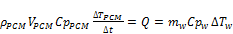(1)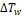: the heat transfer fluid temperature difference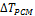: the phase change material temperature difference.

The heat balance for the heat exchanger tube in the tank can be as follows ;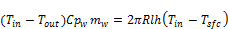(2)

Where the heat transfer coefficient is approximated as ;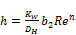(3)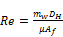(4)

Charging phase:

During the charging phase the water (HTF) mass flow rate can be calculated from the heat released by the solar radiation,

Mass flow rate of water: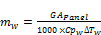(5)

Equation (2) with the finite difference formulation of the time derivative can be written for the solid phase as follows (Figure. 2);

Solid phase: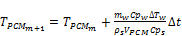(6)

Where: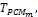Temperature of PCM at m element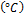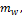Water mass flow rate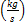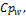Specific heat of water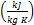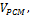PCM volume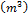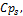PCM specific heat at solid phase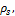Density of PCM at solid phase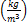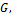Radiation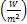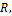Tube radius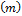Tube length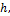Heat transfer coefficient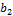&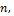Constants equal to 0.3 and 0.6 respectively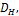Hydraulic diameter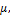Water viscosity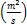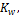Thermal conductivity of water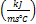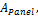Area of solar panel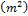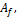Flow area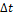: time interval in the finite difference formulation,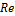: Reynolds number

Same finite difference formulation of the time derivative can be applied to the mushy and liquid phases as follows;

Mushy phase:

The liquid fraction is calculated from the heat balance at the mushy phase region as follows;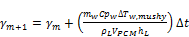(7)

Where the heat absorbed during the mushy phase change is given by;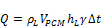(8)

Where,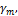Liquid fraction at m element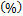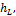PCM latent heat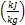Volume of PCM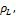Density of PCM at liquid phase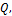Heat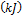Liquid phase:

With the finite difference formulation of the time derivative, the PCM liquid temperature can be calculated as;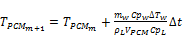(9)

Therefore, the total heat absorbed during the charging process by the phase change material during solid, mushy and liquid phases is;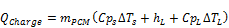(10)

Discharge phase:

During the discharge process, phase change material experiences phase change from liquid to mushy and solid while yielding heat absorbed during the charging process. The water mass flow rate of heat transfer fluid during the discharge process can be calculated by;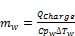(11)

Where;: heat transfer fluid temperature difference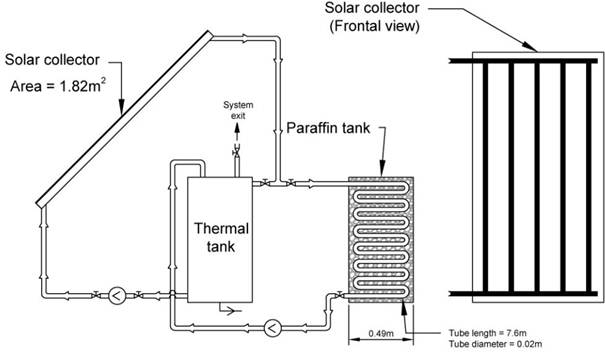Figure 1. Schematic diagram of thermal solar panel and thermal tank system with paraffin.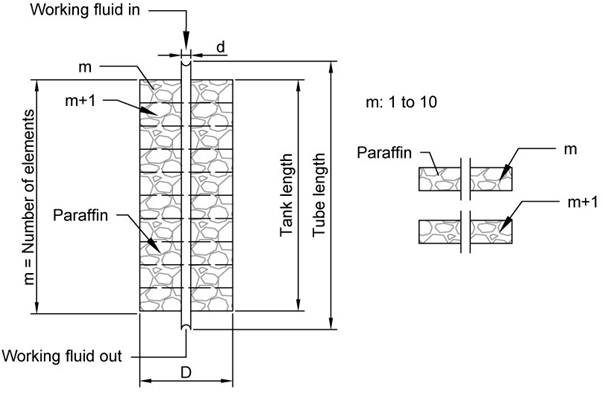Figure 2. Detailed formulation of finite-difference scheme for the thermal solar tank with paraffin.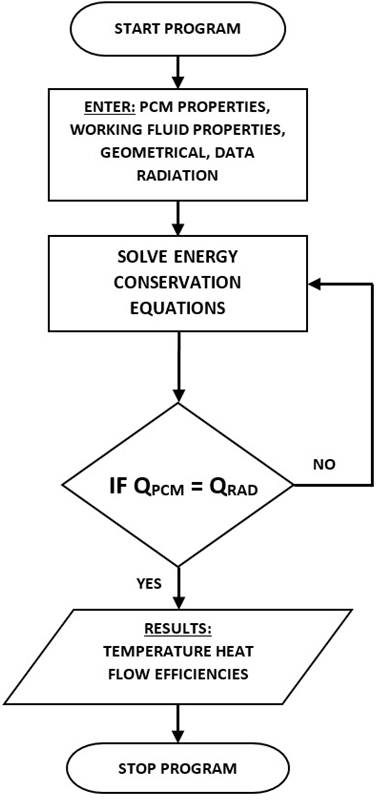Figure 3. Logical flow diagram for finite difference scheme.

3. Numerical Procedure

The energy conversion and heat transfer taking place during charging and discharging periods for phase change material paraffin wax placed in the thermal solar tank have been outlined in equations (1) through (11). The aforementioned equations have been solved as per the logical flow diagram shown in Figure.3, where the input independent parameters are defined and other dependent parameters were calculated and integrated in the finite-difference formulations. Iterations were performed until a solution is reached with acceptable iteration error. The numerical procedure starts with the use of the solar radiation to calculate the mass flow water circulating in the solar panel. This follows by predicting the temperature profile of the phase change material and heat transfer fluid during the charging and discharging periods for the three phases; solid, mushy and liquid using the finite difference formulation.

4. Discussion and Analysis

The aforementioned system of equations (1) through (11) in finite-difference forms have been integrated and numerically solved and samples of the predicted results are plotted in Figures 5 through 13, at different inlet conditions.

The thermal tank has diameter of 0.49 meter and height 0.99 meter with capacity of 100 liters and one path tube heat exchanger of 0.025 meter and 7.5-meter length. The heat transfer fluid; water flows inside the aforementioned one path tube heat exchanger as shown in Figures. 1 and 2. Three PCMs paraffin waxes are considered in the present simulation with their thermal and thermophysical properties as presented in Table. 1. Paraffin waxes were selected because they are the most commonly used commercial organic heat storage PCM due to their large latent heat and moderate thermal energy storage density, low vapor pressure, good thermal and chemical stability, lack of thermal separation and environmentally sound .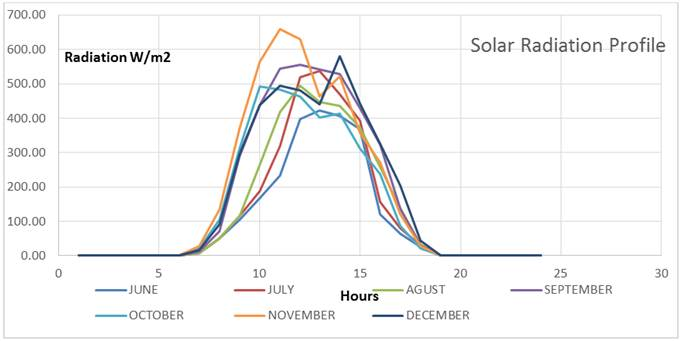Figure 4. Time variation of solar intensity.

In general, it is quite clear from figures (5) through (13) that initially the phase change material (paraffin wax) temperature increases during the solid phase until reaches the fusion temperature (melting point) where the temperature stays constant until the onset of the liquid phase where the temperatures increases until reaches the charging temperature that is determined by the heat transfer fluid conditions. Therefore, during this process, the phase change material experiences sensible heat addition during the solid and liquid phases and latent heat addition during the mushy phase. In the following sections, we present simulation results of different paraffin wax phase change material at different conditions during the charging and discharging process. Thermodynamic and thermophysical properties of the paraffin wax materials are presented in Table. 1.

In particular, Figure. 4 presents the time variation of solar insolation (W/m2) measured at the site and employed in the simulation. It is quite clear that the intensity of radiations depends upon the hour of the day and the month of the year.

Figure 5 displays the predicted behavior of phase change material paraffin wax  during the charging process for a cycle of 8 minutes under constant solar radiation of 550 W/m2. This figure presents the temperature profile of the heat transfer fluid and consequent temperature increase of the paraffin wax. Such a behavior was presented during the solid phase and fusion (mushy) phase. It can be observed that the heat transferred from the HTF to paraffin wax caused the paraffin wax’s temperature to rise and the HTF’s temperature to decrease. Furthermore, Also Figures. 5 through 7 show the same behavior for three paraffin waxes  under investigation at different solar radiations. As can be seen from the figures, the charging time can vary depending upon the properties of each paraffin wax, namely, the melting temperature, and specific heat among other properties as well as the conditions of the heat transfer fluid properties.

In particular, Figure. 6 and 7 display the temperature time variation of paraffin 1, 2 and 3 under different solar intensity radiations. It is quite clear from the results presented in these figures that the initial paraffin temperature increases during the charging process until reached the melting temperature and the threshold of the mushy phase, where the paraffin temperature remains constant until the end of the mushy/latent heat phase. As shown in the figures, the paraffin temperature starts to increase until the end of the charging phase where the paraffin is fully converted into liquid. It is worthwhile noting that this behavior has been observed for the three paraffin waxes.

On the other hand, figures 8 and 9 present the time variation of the heat absorbed during the charging process for three paraffin waxes under different solar radiation. The results clearly show that sensible heats were observed during the solid and liquid phase where heat was increased similar to the temperature time variation presented in the previous figures. Latent heat was constant in the mushy phase where the melting temperature is constant. Furthermore, the results also showed that the higher the solar intensity radiation the higher the heat absorbed. Similar behavior has been observed for the other paraffin waxes.

Figure.10 illustrates the temperature time variation of the paraffin wax and the heat transfer fluid where the liquid phase material is converted into mushy and solid at the end of the discharging process. The paraffin temperature decreases during the discharging process due to the heat transferred to the heat transfer fluid. The amount of the heat transferred depends upon the final condition of the paraffin wax by the end of the charging process and the heat transfer fluid thermal and thermophysical properties.

Figures 11and 12 present the temperature profiles during discharging process for paraffin waxes PCM2 under different solar intensity radiation. Obviously, the amount of heat transferred to the heat transfer fluid is dependent upon the thermophysical and thermodynamic properties of the paraffin wax [Table.1]. As discussed previously, and similar to the behavior observed of the paraffin waxes during the charging process, the discharging time has a functional dependence upon the type of paraffin wax. On the other hand, Figure.12 illustrates that the discharging characteristics of paraffin wax depend also upon the solar radiation intensity used during the charging process and the higher the radiation the lower charging time and consequently the shorter discharging time. Clearly, the required energy to raise the water temperature is influenced by solar radiation intensity.

Furthermore, Figure.13 shows that the amount of heat released from the paraffin waxes to the heat transfer fluid is impacted by thermal and thermophysical properties of the phase change material. As shown in Figure 13, the amount of heat released is dependent upon the final condition by the end of the charging process and the heat transfer fluid conditions. Similar behavior was observed under different solar radiation intensity.

Figure.14 and 15 have been constructed to show the impact of heat transfer fluid conditions on the heat released by paraffin during charging and discharging process at different water flow rates and constant solar radian intensity. It is evident from results displayed in these figures that higher water flow rate yields to higher heat released and consequently higher paraffin and water temperatures during the charging and discharging processes, respectively. In addition, similar behavior was observed at other solar isolations.

From the aforementioned discussion, it is worthwhile noting that in sensible heat storage, thermal energy transferred from or to the heat transfer fluid is stored by raising the temperature of a solid or liquid. The solar and phase change material system, in question, utilizes the heat capacity and the change in temperature of the material during the process of charging and discharging. However, the latent heat storage is based on the heat absorption or release when a storage phase change material undergoes a phase change from solid to liquid or liquid to solid.

In order to validate the proposed model to predict the behavior of phase change material in a solar thermal tank, experimental data presented by Fazilati, and Alemrajaki,  have been simulated during the charging and discharging process have been presented in Figures 16 and 17. It is quite clear from the results presented in Figure.16 that the proposed model fairly predicted the data of time variation of the paraffin wax temperatures during the charging process and the sensible heat periods. The slight difference between the data and model prediction suggest that there some heat losses during the latent heat region which was not taken into account fully by the model formulation.

However, Figure 17 presents the comparison between the model’s prediction and the paraffin data during the discharging process. It appears from this figure that the model over predicted the data in the solid phase during the discharging process. This was attributed to heat losses that were not taken into account in the model.

Finally, Figure 18 has been constructed to compare the thermal storage energy conversion efficiencies of different paraffin waxes. In this figure, the efficiencies of seven paraffin waxes were presented; paraffin-1 , paraffin-2 , paraffin-3 , paraffin-4 through-7 . The energy conversion efficiency is defined as the ratio between the discharge heat to the charging heat of paraffin wax. As discussed in the aforementioned sections, the energy conversion thermal storage efficiency mainly has a functional dependent upon the thermodynamic and thermophysical properties of paraffin wax. The results presented in this figure also show that the higher the efficiency the lower the melting point and the ability to discharge more heat to the heat transfer fluid. In addition, it is worthwhile mentioning that higher melting temperature requires higher thermal energy during the charging process. However, in some special applications, higher melting temperatures are preferred if higher discharging temperature is needed and energy conversion efficiency is compromised.

Table 1. Paraffin wax properties.

 Paraffin Wax 1  Melting point 46.7°C Specific heat (solid) 2.89 kJ/kg°K Specific heat (liquid) 2.89 kJ/kg°K Density (solid) 947 kg/m3 Density (liquid) 750 kg/m3 Latent heat 209 kJ/kg Paraffin Wax 2  Melting point 41°C Specific heat (solid) 2.48 kJ/kg°K Specific heat (liquid) 2.76 kJ/kg°K Density (Solid) 829 kg/m3 Density (Liquid) 765 kg/m3 Latent heat 288 kJ/kg Paraffin Wax 3  Melting point 37°C Specific heat (solid) 1.82 kJ/kg°K Specific heat (liquid) 2.17 kJ/kg°K Density (solid) 911 kg/m3 Density (liquid) 799 kg/m3 Latent heat 201 kJ/kg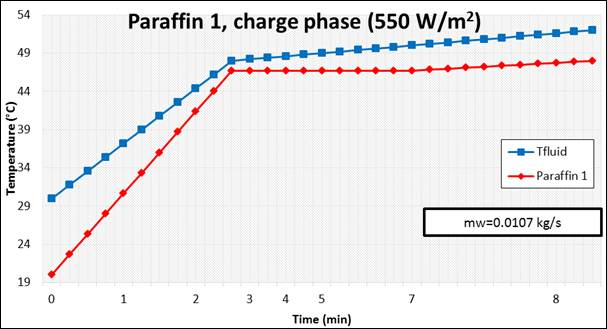Figure 5. Temperature profile during charging phase under solar radiation 550 W/m2.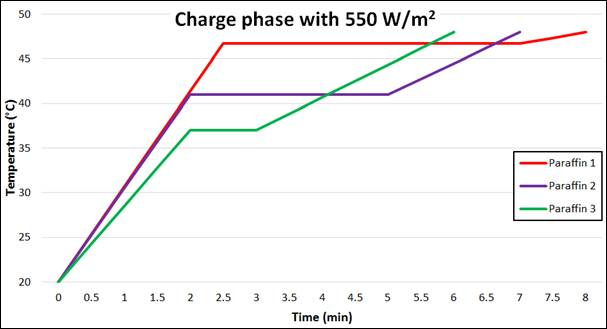Figure 6. Comparison of different paraffin wax [Table.1] under different solar radiations.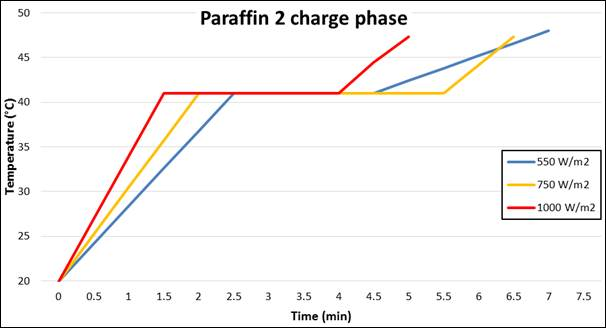Figure 7. Behaviour of paraffin 2 [Table.1] under different solar radiations.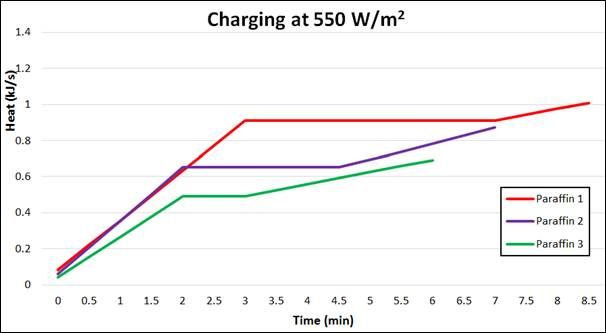Figure 8. Heat absorbed by different paraffin wax [Table.1] under constant solar radiation.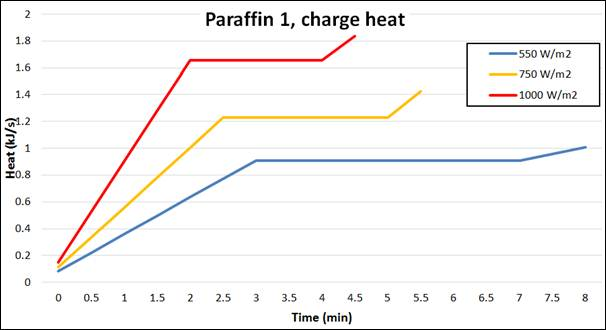Figure 9. Heat absorbed by paraffin 1 [Table.1] under two different solar radiations.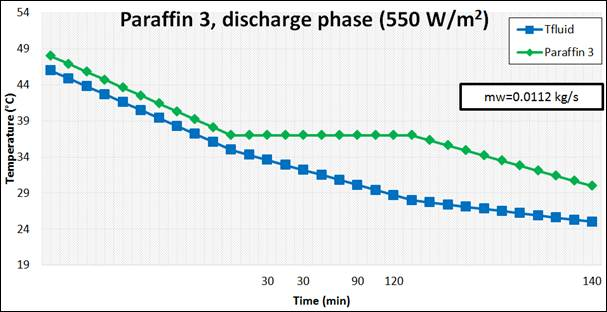Figure 10. Behaviour of paraffin wax [Table.1] during discharging process.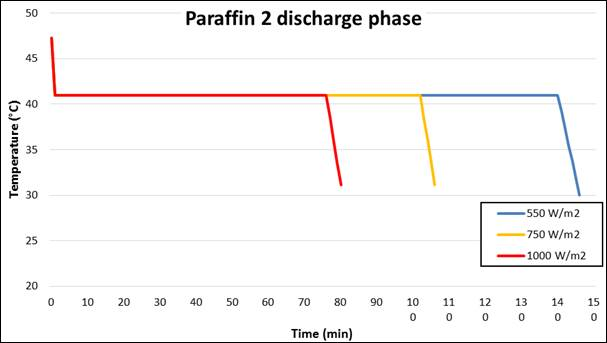Figure 11. Paraffin wax 2 [Table.1] during discharging process while charging under different solar radiations.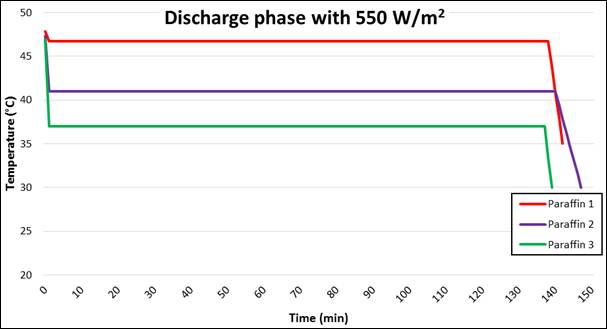Figure 12. Temperature time variation during discharging by different paraffin wax [Table.1] under solar radiation 550 W/m2.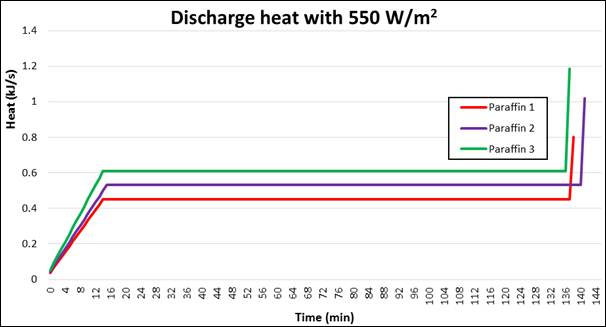Figure 13. Heat released during discharging by different paraffin wax [Table.1] under solar radiation 550 W/m2.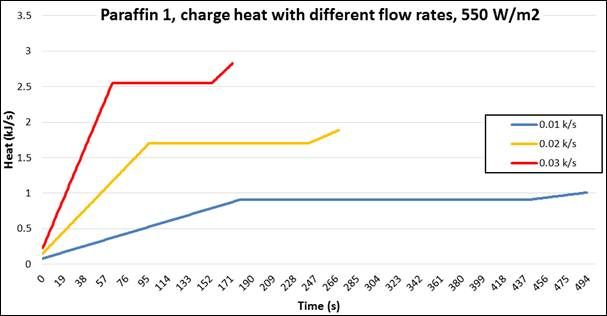Figure 14. Heat released by paraffin wax 1 [Table. 1] at different HTF mass flow rates during discharging process.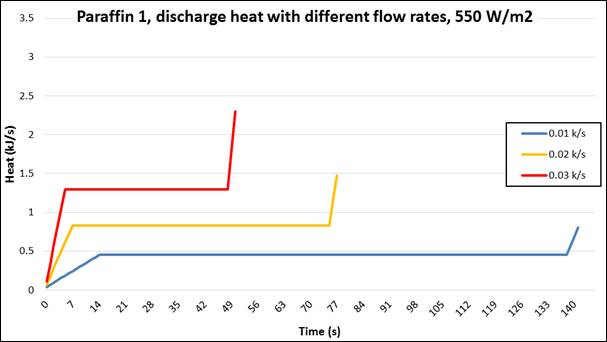Figure 15. Heat released by paraffin wax 1 [Table. 1] at different HTF mass flow rates during discharging process.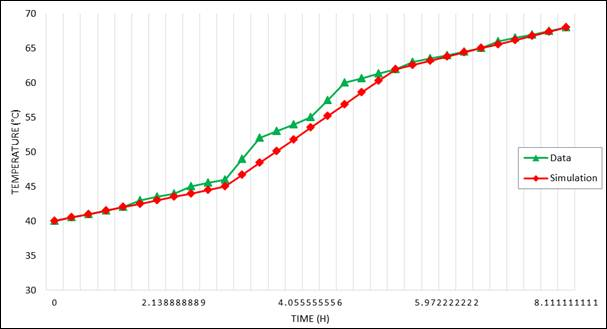Figure 16. Comparison between model prediction of charging paraffin wax and data .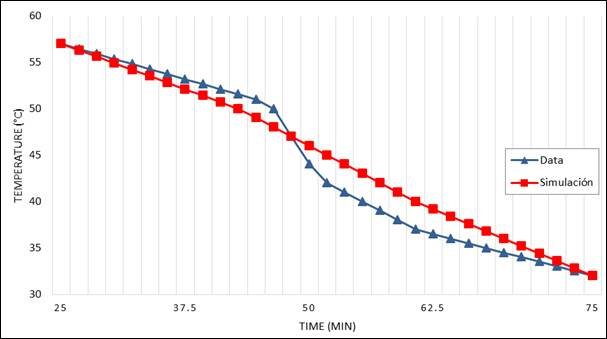Figure 17. Comparison between model prediction and data  during discharging process.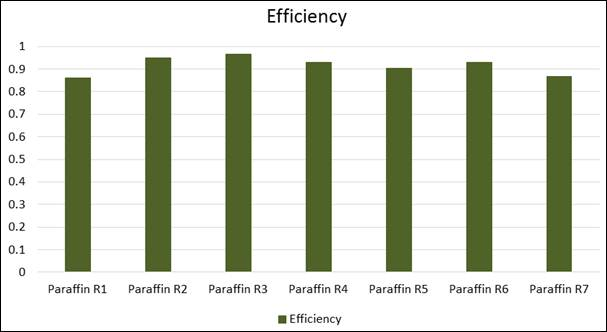Figure 18. Energy Conversion Efficiency comparison between different paraffin waxes.

5. Conclusions

During the course of this study, the phase change material characteristics during the charging and discharging processes of paraffin wax in solar thermal tank have been modeled, presented and analyzed. The model was established after the energy conservation equations coupled with the heat transfer equations. In general, the presented numerical model fairly predicted the phase change material heat transfer characteristics during charging and discharging processes and compared well with the experimental data.

Nomenclature

APanel, Area of solar panel (m2)

Af, Flow area (m2)

Cps, PCM specific heat at solid phase (kJ/(kg K))

Cps, PCM specific heat at solid phase (kJ/(kg K))

Cpw, Specific heat of water (kJ/(kg K))

DH, Hydraulic diameter (m)

h, Heat transfer coefficient

hL, PCM latent heat (kJ/kg)

Kw, Thermal conductivity of water (kJ/(ms°C))

l, Tube length (m)

mw, Water mass flow rate (kg/s)

N: number finite different element (N: 1-12)

Qtub, Heat (kJ)

TPCM, m, Temperature of PCM at "m" element (°C)

VPCM, PCM volume (m3)

VPCM, Volume of PCM (m3)

Greek

ρs, Density of PCM at solid phase (kg/m3)

ρL, Density of PCM at liquid phase (kg/m3)

μ, Water viscosity (m2/s)

γm, Liquid fraction at m element (%)

Subscripts

H: Hydraulic

L: Liquid

PCM: Phase change material

S: Solid

Tub: tube

W: Water

Acknowledgement

The research work presented in this paper was made possible through the support of the Catholic University of Cuenca.

References

1. Tian, Y, and Zhao, CY A review of solar collectors and thermal energy storage in solar thermal applications. Applied Energy 104 (2013): 538–553.
2. Farid, M. M, Khudhair, A. M., Razack, S. A. and Al-Hallaj S. A review on phase change energy storage material. Energy Conversion and Management, 2004; 45: 1597.
3. Elawadhi, E. M. Phase change process with free convection in a circular enclosure; numerical simulation. Computer & Fluids, 2005; (33), 1335-148.
4. Tardy, F. and Sami, S. (2008), An experimental study determining behaviour of heat pipes in thermal storage, International Journal of Ambient Energy, 2008; 29, (3).
5. Razali, T, Hamdani, Irwasnsyah, Zaini, (2004), Investigation of performance of solar water heater system using paraffin wax, ARPN Journal of Engineering and Applied Sciences, 2014; 9, (10).
6. Thirugnanm, C, and Marimuthu, P (2013), Experimental analysis of latent heat thermal energy storage using paraffin wax as phase change material, International Journal of Engineering and Innovative Technology (IJEIT), 2013; 3, (2).
7. Hale DV, Hoover MJ, O’Neill MJ. (1971), Phase change materials hand book. Alabaa: Marshal Space Flight Center.
8. Sami, S., and Tardy, F. (2015),Numerical prediction of thermal storage using phase change material" IJIRE, Volume 3, No 4.
9. Ukrainczyk, N., Kurajica, S., and Sipusic, J, (2010), Thermophysical comparison of five commercial paraffin waxes as latent heat storage materials, Chem. Biochem. Eng. Q 24, (2), 129-137.
10. Khot, S. A., (2014), Enhancement of thermal storage system using phase change material, Energy Procedia, 54, 142-151, 2014.
11. Lin, S. C, Al-Kayiem, H. H. and Bin Aris, M., S., (2012), Experimental investigation on performance enhancement of integrated PCM-Flat solar collector, Journal of Applied Sciences, 12,(23), 2390-2396, 2012.
12. Kanimozhi, B, and Bapu, R., (2012), Experimental study of thermal energy storage in solar system using PCM, Transaction on Control and Mechanical Systems, vol. 1,No.2, Pp. 87-92, 2012.
13. Kulkarni, M. V. and Deshmulkh, D. S., (2014), Improving efficiency of solar water heater using phase change materials, IJSSBT, vol. 3, No.1, Dec. 2014.
14. Milisic, E, (2013), Modeling of energy storage using phase-change materials (PCM materials), Master thesis, Norwegian University of Science and Technology, July 2013.
15. Mahmus, A, Sopian, K, Alghoul, M. A. and Sohif, M, (2009), Using a paraffin wax-aluminum cpound thermal storage material in solar air heater, ARPN Journal of Engineering and Applied Sciences, Vol. 4, No.10, pp. 74-77, Dec 2009.
16. Fazilati, M. A. and Alemrajaki, A. (2013), Phase change material for enhancing solar water heater, an experimental approach, ECM Energy Conversion and Management, Vol. 71, pp.138-145, 2013.
17. Razali, T. et al. (2014). Investigation performance of solar water heater system using paraffin wax. ARPN Journal of Engineering and Applied Sciences, Vol. 9, 1749-1752.
18. Novak, P. et al. (1996). Thermal storage of solar energy in the wall for building ventilation. Renewable Energy, Vol. 8, Issue 1-4, 268-271.
19. Fortunato, B. et al. (2012). Simple Mathematical Model of a Thermal Storage with PCM. ELSEVIER, Procedia 2, 241-248.
20. Ukrainczyk, N., et al. (2010). Thermophysical Comparison of Five Commercial Paraffin Waxes as Latent Heat Storage Materials. ChemBioChem, Vol. 24, 129-138.

 Contents 1. 2. 3. 4. 5.
Article ToolsAbstractPDF(8987K)